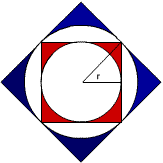#### You may also like### Polycircles

Show that for any triangle it is always possible to construct 3 touching circles with centres at the vertices. Is it possible to construct touching circles centred at the vertices of any polygon?### Nim

Start with any number of counters in any number of piles. 2 players take it in turns to remove any number of counters from a single pile. The loser is the player who takes the last counter.### Loopy

Investigate sequences given by $a_n = \frac{1+a_{n-1}}{a_{n-2}}$ for different choices of the first two terms. Make a conjecture about the behaviour of these sequences. Can you prove your conjecture?

# Squaring the Circle and Circling the Square

##### Age 14 to 16 Challenge Level:

Many thanks to Andrei Lazanu of Bucharest for the following solution.

I know that the area of the circle of radius $r$ is $\pi r^2$.

##### Part 1

1) Here, the side of the red square is $2r$.

The area of the square is $4r^2$ .

So, subtracting the area of the circle, I obtain the area of the red sector:

$$4r^2 - \pi r^2 = r^2(4-\pi)$$

2) Here I look at the diagonal of the red square, that is half the side of the blue square:I look at the right-angled triangle, with the hypotenuses half the diagonal of the red square, and two sides of lengths equal to the radii of the first (interior) circle. It is also an isosceles triangle. The radius of the big circle is the hypotenuses of the described triangle. Its length is $r \sqrt{2}$.

Now, the side of the blue square is $2r \sqrt{2}$ , and its area is $8r^2$ .

The area of the big circle is $2 \pi r^2$.

The area of the blue portion is:
$$8r^2 - 2\pi r^2 = 2r^2(4-\pi)$$

3) Here, I observe that I need to calculate the radius of the biggest circle, by multiplying the previous result by $\sqrt{2}$ obtaining $2r$. The area of the orange square is $16r^2$ , and of the circle $2\pi r^2$.

Now, the area of the orange portion is

$$16r^2 - 4\pi r^2 = 4r^2(4-\pi)$$

4) Now I look at the general case: there are n circles, the first having the radius $r$. The radius of the $n$-th circle will be

$$r(\sqrt{2})^{n-1}$$

The area of the corresponding square is:

$$4 r^2 \left(\sqrt{2}\right)^{2(n-1)}=4 r^2 2^{n-1}=2^{n+1}r^2$$

and of the corresponding circle:

$$\pi r^2\left(\sqrt{2}\right)^{2(n-1)}= \pi r^2 2^{n-1} = 2^{n-1} \pi r^2$$

$$2^{n+1}r^2 - 2^{n-1}\pi r^2 = 2^{n-1}r^2 (4-\pi)$$

which has as particular cases exactly what I obtained before.

##### Part 2

For this part of the problem, I must go back to smaller and smaller radii of circles: I have to divide each time by $\sqrt{2}$ to obtain the new radius. So, for the $n$-th square in the image, the blue part will be:

$$r (\sqrt{2})^{n-1}$$

I know that I started from the first square to the $n$-th, so the sum is:

\begin{eqnarray}S&=& \frac{r^2 (4-\pi)}{2^{1-1}}+\frac{r^2 (4-\pi)}{2^{2-1}}+\frac{r^2 (4-\pi)}{2^{3-1}}+ \ldots +\frac{r^2 (4-\pi)}{2^{n-1}}\\ &=&\frac{r^2 (4-\pi)}{2^0}+ \frac{r^2 (4-\pi)}{2^1}+\frac{r^2 (4-\pi)}{2^2}+ \ldots +\frac{r^2 (4-\pi)}{2^{n-1}} \\ &=& r^2 (4-\pi)\left(1+\frac{1}{2}+\frac{1}{2^2}+\ldots+\frac{1}{2^{n-1}}\right)\end{eqnarray}

I simplify each fraction from the sum with a corresponding value, so that the denominator would be $2^{n-1}$ :

$$S = r^2(4-\pi)\left(\frac{1+2+2^2+\ldots+2^{n-1}}{2^{n-1}}\right)$$

The numerator is a geometric progression with common ratio $2$ and $n$ terms.

$$S=r^2(4-\pi)\left(\frac{2^{n}-1}{2^{n-1}}\right)$$

But, in this case $n$ is infinite, so

$$\frac{1}{2^{n-1}}$$

tends to zero. So,

$$S = r^2(4-\pi)\frac{2^n}{2^{n-1}}= 2r^2 (4-\pi)$$